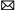inquiry@ato.com.cn
+1 800-585-1519 (Toll-free)

Home » Wiki » Regulator Circuit for Multi Load Cells in Parallel Connection

# Regulator Circuit for Multi Load Cells in Parallel Connection

The medium-sized and large electronic scales are made up of several strain gauge load cells (hereinafter abbreviated as the 'load cell'). There is only one meter for weight display. Several load cell group scales are connected through two means, either group-scale in series or group-scale in parallel. Due to rapid development of electronic technologies and popularization of SCM, weighing instruments have experienced a significant improvement of output sensitivity, which can fully satisfy the technological requirements of the group-scale in parallel. Therefore, the group-scale in series has been eliminated, and the group-scale in parallel is more widely adopted.
However, affected by civil construction and mechanical installation, the electronic weighing instrument can easily cause uniform distribution of force on various load cells. Besides, because of manufacturing differences of load cells, various parameters cannot ensure their consistency despite of circuit compensation and adjustment. As a result, unacceptable eccentric error is caused for the electronic balancing instrument with multi load cells working in parallel connection. Whether the eccentric load test is qualified or not in the electronic weighing instrument industry is a key item for whether the electronic weighing instrument can pass the test and be used normally. Therefore, it is necessary to explore an effective and reasonable debugging method to reduce the eccentric error by analyzing the eccentric load debugging principle of load cell parallel group-scale.

I. Brief analysis of load cell parallel circuit
The load cell is the bridge circuit formed by regular pasting of resistance strain gages on the elastic element. In order to ensure multi technical parameters to reach the requirements, it is necessary to conduct circuit compensation and adjustment one by one. Meanwhile, the compensating resistance should be added. See figure 1 below: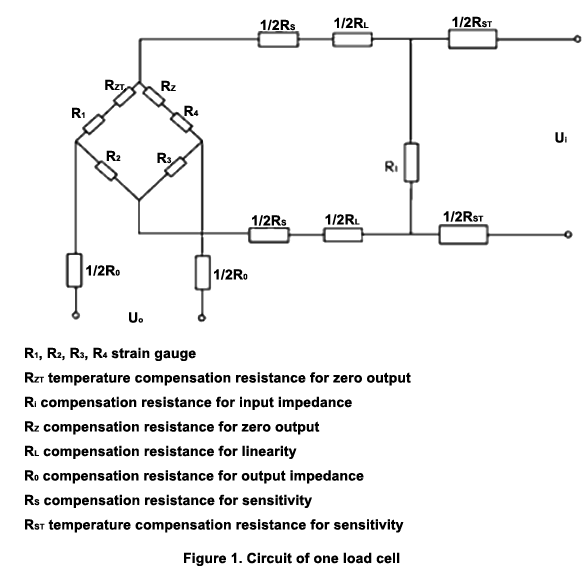According to Thevenin's theorem, the circuit of a load cell is equal to the circuit of the series connection between a voltage source and its output resistance. See figure 2 below: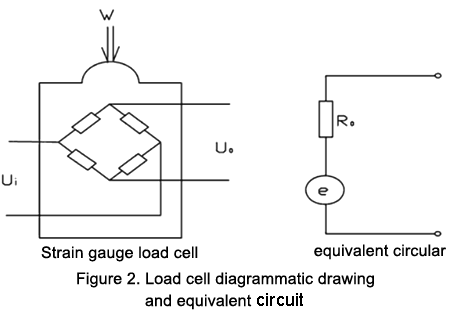e is the voltage signal generated by the load cell after loading. Its value is in direct proportion to the sensitivity coefficient, K, and weight, W, of the load cell.
RO is the output resistance of the load cell. If the output terminal of the load cell is connected with an adjustable potentiometer, RW, the output voltage of multi load cells is the same, that is, U01=U02=U0n. See figure 3 below. The most commonly-used eccentric load adjustment method is based on this principle.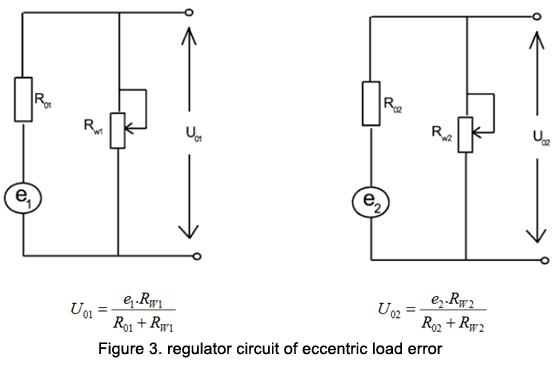2. Circuit of multi load cells connected in parallel
The circuit of multi load cells connected in parallel is shown in figure 4 below. The input terminal of multi load cells is connected in parallel. The direct current stabilizing power source of the load meter is adopted as the bridge and the output terminal is also connected in parallel. The signal voltage is output to the load cell controller.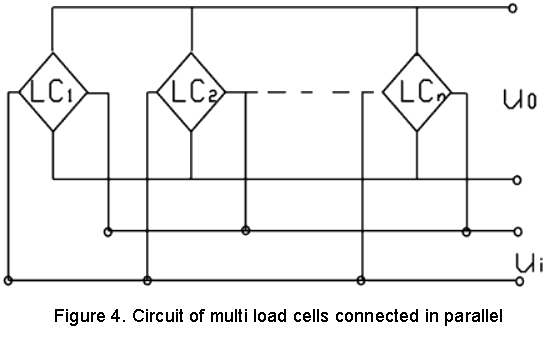The equivalent circuit output by n load cells connected in parallel is shown in figure 5.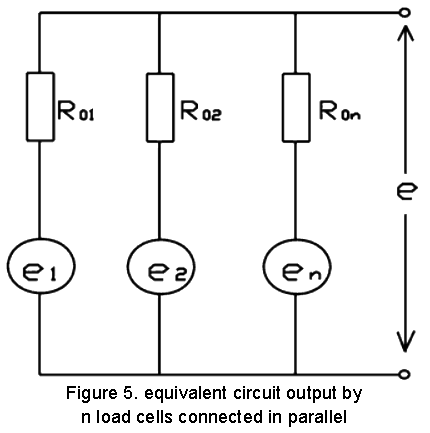ei is the output voltage of the output terminal open circuit of the i load cell.
R0i is the output resistance of the voltage source short circuit of the i load cell.
e is the total output voltage of n load cells after parallel connection.
The node voltage equation for the equivalent circuit output by parallel connection can be written as below:
e(1/R01+1/R02+...+1/R0n)=e1/R01+e2/R02+...+en/R0n
As ei=kiwi, so equation of e is as follows: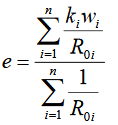From the above equation, it can be seen that, in the electronic weighing instrument made up of n load cells connected in parallel, ∑=niiR101, must be a certain constant, which will not cause eccentric load to the electronic weighing instrument. However, dispersion of Ki and R0i will directly influence the proportional relationship between e and w.
In other words, only when the sensitivity coefficient, Ki, and the output resistance, R0i, of multi load cells are the same, or their ratio is equal to iiRK0 is the same can the value displayed by the same load, W, at different positions of the electronic weighing instrument platform be the same, which is actually no eccentric load. Obviously, a necessary condition for multi load cell parallel-connection group-scale is the iiRK0 value of various load cells should be consistent. However, dispersiveness of the sensitivity coefficient and output resistance of load cells of the same series and the same specifications turned out by mass production is inevitable. Besides, coupled with civil construction and mechanical installation factors, the wiring adjustment box should be set for the multi load cell parallel-connection group-scale to cope with the problem of eccentric load.

II. Practical regulator circuit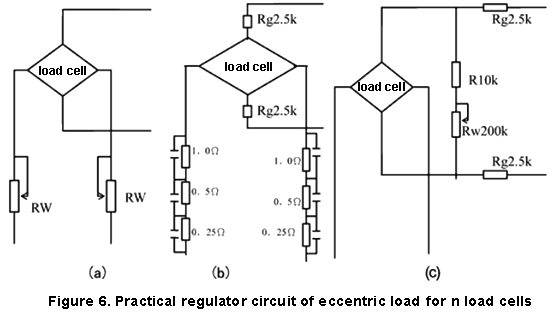In parallel-connection of group-scales in the load cell, the commonly-seen eccentric load regulator circuit is shown in figure 6 below. As known to all, compensation and modification of various technological parameters during manufacturing of load cells is implemented by symmetric adjustment. For example, the sensitivity coefficient adjustment resistance is connected to two sides of the input end in a symmetric form. The so-called voltage adjustment is in essence fine-tuning of the sensitivity coefficient, which should also appear on the input terminal symmetrically. Figure 6 (a) is to connect a pair of precision potentiometers (RW=20Ω) in series to the input end of multi load cells. Through fine adjustment of the excitation voltage of multi load cells, the sensitivity coefficient can be changed so that the Ki/Roi ratio value of various load cells is consistent with each other which can effectively eliminate the eccentric error.
Figure 6 (b) is the circuit in the wiring box on the load cell. First, connect a pair of precision resistors with the error of 0.05% (2.5K~5k) to the output terminal of multi load cells. The precision resistance value is far larger than the output resistance of the load cell. In this way, the dispersion degree of the load cell output resistance can be effectively reduced. Second, connect three precision resistors to two ends of the input terminal symmetrically. Every resistor has the short welding point for adjustment. This is actually to change the value of the excitation voltage of every load cell and conduct fine-tuning of the sensitivity coefficient to achieve consistency of Ki/Roi of various load cells. The practical situations suggest that the adjustment method shows a long-term stability compared with the potentiometer. See Figure 6 (a). In using the above two adjustment methods, attention should be paid to symmetry on two sides. In the early period, though some regulator circuits just needed to connect one precision potentiometer to one side of the input terminal in series, difference of the central-point potential of the load cell can be easily caused, thus impairing the precision of output zero points and weighing precision, which is not promoted.
Figure 6 (c) is a regulator circuit commonly adopted by the group-scale constituted of multi load cells connected in parallel. The resistor, R, is set up to prevent short circuit of the potentiometer, Rw. The precision resistor, Rg, is an isolation resistance, which can separate the voltage apart after regulation of various load cells. The output voltage of the load cell, after undergoing the isolation resistance, is connected in parallel and sent to the weight instrument. Obviously, by changing Rw, the output voltage of various load cells can be consistent. Besides, the isolation resistance can also reduce the dispersion degree of the load cell output resistance and eliminate the eccentric error. The actually used wiring is shown in Figure 7. (The TVS pipe is not drawn.) This method has been widely accepted.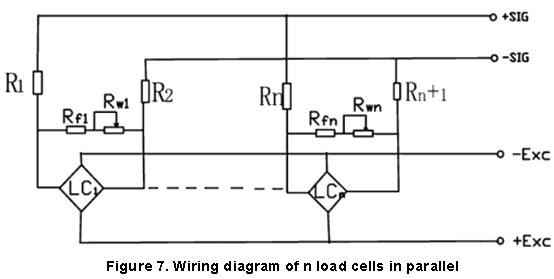Under the condition of not considering the influence of other load cells in parallel connection, the equivalent circuit is drawn. Take the load cell with the output resistance of RO=700 for example. The regulating scope of the following equivalent circuit is -0.3%～-6.5%, and the adjusting quantity is 6.2%.

I have an Opinion:

Plz Calculate (3 * 2) =
(Your comment will show after approved.)

You may also like: